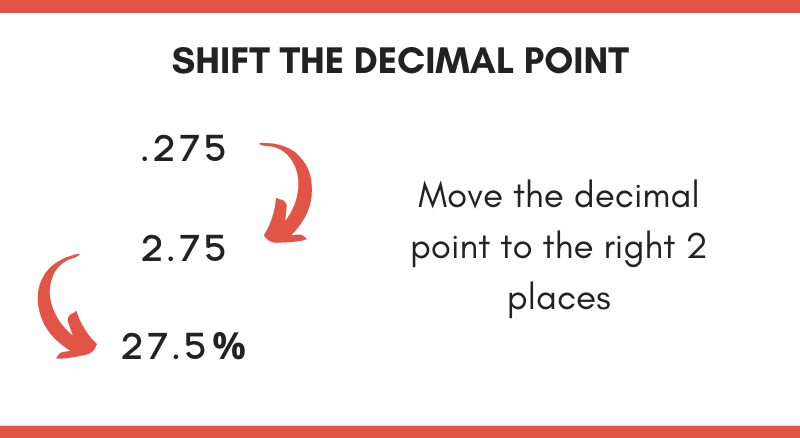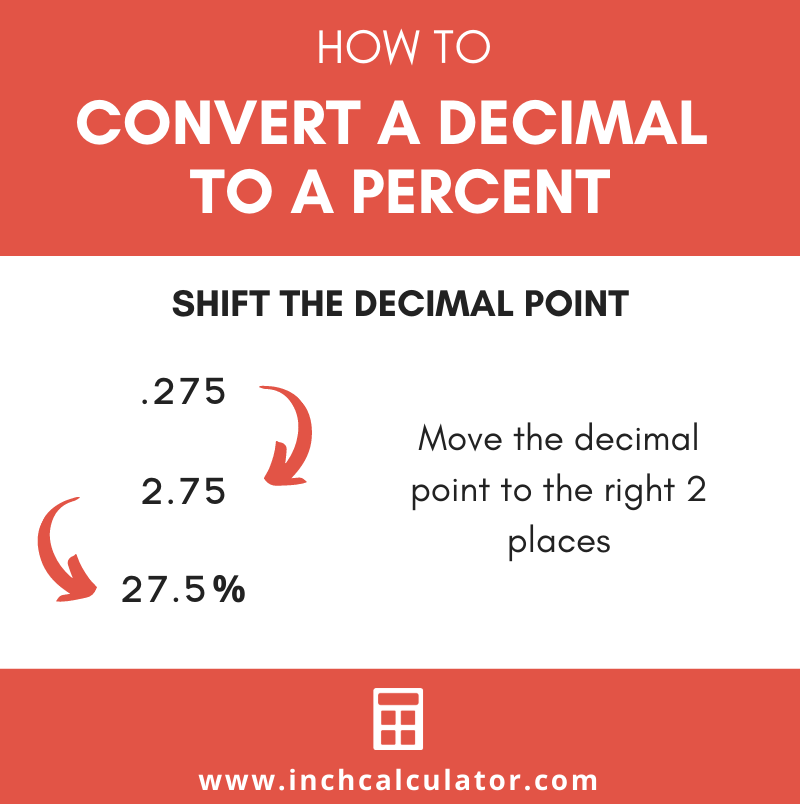# Decimal to Percent Calculator

Convert a decimal to percent by entering the decimal value in the calculator below.

## Percentage:

75%

### Steps to Convert a Decimal to Percent

p=decimal×100
Substitute value in the equation
p=0.75×100
Solve
p=75%
Learn how we calculated this below

Do you want to convert a percent to decimal?

## How to Convert a Decimal to a Percent

Percent means “per 100” and can be expressed in percent form which has a % symbol after the number, or in decimal form, which does not have the % symbol.

To convert from a decimal number to percent form, simply multiply the decimal by 100 and add the % symbol to the end. See the following formula for converting a decimal to a percentage.

p = d × 100

Thus, the percentage p is equal to the decimal d times 100.

For example: let’s convert .367 to a percent using the formula.

p = decimal × 100
p = .367 × 100
p = 36.7%

An alternative approach is to simply move the decimal point to the right 2 places.

For example: let’s convert .275 to a percent by shifting the decimal place to the right 2 places.

p = .275
p = 2.75
p = 27.5%Try our percentage calculator to solve more percentage problems.

## Decimal to Percent Conversion Chart

Another way to convert a decimal to percent is to use a conversion table. The table below shows common decimal values and their equivalent percentage values.

Decimal to percent conversion chart showing numbers and the equivalent percentage
Decimal Percent
.001 .1%
.002 .2%
.003 .3%
.004 .4%
.005 .5%
.006 .6%
.007 .7%
.008 .8%
.009 .9%
.01 1%
.015 1.5%
.02 2%
.025 2.5%
.03 3%
.035 3.5%
.04 4%
.045 4.5%
.05 5%
.055 5.5%
.06 6%
.065 6.5%
.07 7%
.075 7.5%
.08 8%
.085 8.5%
.09 9%
.095 9.5%
.1 10%
.15 15%
.2 20%
.25 25%
.3 30%
.35 35%
.4 40%
.45 45%
.5 50%
.55 55%
.6 60%
.65 65%
.7 70%
.75 75%
.8 80%
.85 85%
.9 90%
.95 95%
1 100%
2 200%
3 300%
4 400%
5 500%
6 600%
7 700%
8 800%
9 900%
10 1000%